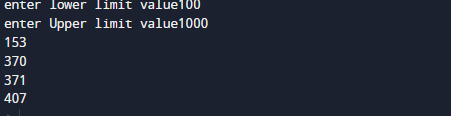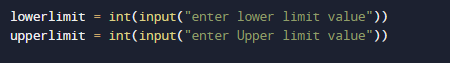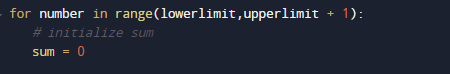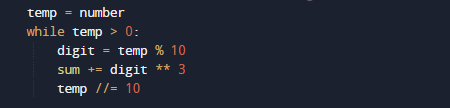# Python Program to Find Armstrong Number in an Interval

In this tutorial you will learn about the Python Program to Find Armstrong Number in an Interval and its application with practical example.

In this tutorial, we will learn to create a Python program to Find Armstrong Number in an Interval using Python programming.

## Prerequisites

Before starting with this tutorial we assume that you are best aware of the following Python programming topics:

• Basic Input and Output
• Basic Python  programming.
• Python if-else statements.
• Python Loop.

## What Is Armstrong Number?

A number is called An Armstrong  number in which the sum of the cube of the individual digits in number  is equal to the number itself.
For example 0, 1, 153, 370, 371 and 407 are the Armstrong numbers. Let’s try to understand why 153 is an Armstrong number.

=>407 = ((4*4*4)+(0*0*0)+(7*7*7) )

= 407 =( 64 + 0 + 343)

=>407 = 407 (So this is an Armstrong number.)

## Python Program to Find Armstrong Number in an Interval

In this program we will find Armstrong number between the given Interval using while loop. We would first declared and initialized the required variables. Next, we would prompt user to input a upper and lower limit range . Later we will find Armstrong numbers.

## OutputIn this program we take lower and upper limit from user  and find Armstrong number between given intervals.

In the above program, we have first declared and initialized a set variables required in the program.

• lowerlimit = it  will hold lower-limit.
• upperlimit = it  will hold upper-limit.
• number= it will hold number value
• temp= for holding remainder
• sum = for adding values.
• Digit= for finding Armstrong number.After taking upper and lower limit from user we will use a for loop to iterate between lower to upper limit and within the range we will find Armstrong number.Here, we take value from user and within the interval we want to search Armstrong numbers. Within the interval we find and display all the Armstrong numbers that meet our condition.With in the Interval we can see that there are 4 three(3) digit Armstrong numbers.

In this tutorial we have learn about the Python Program to Find Armstrong Number in an Interval and its application with practical example. I hope you will like this tutorial.